# Financial Network¶

Author: Erika Fille Legara

You are free to use (or change) this notebook for any purpose you'd like. However, please respect the MIT License that governs its use, and for copying permission.

Copyright © 2016 Erika Fille Legara

## Description¶

I have been receiving requests to release the Python code I wrote to produce the financial network discussed in my blog post at erikafille.ph titled PSE Correlation-Based Network. Here it is.

In this notebook, we build a correlation-based network (minimum spanning tree (MST)) of companies listed in the Philippine Stock Exchange. The entire process can be summarized into three steps:

1. Set up the correlation matrix for the stock prices in the Philippine Stock Exchange.
2. Convert the resulting correlation matrix into a distance matrix.
3. Build a minimum spanning tree from the distance matrix.

### Data¶

I got the link to the data from this stock forum post. In this post it says Drop Box: 2006-present worth of CSV files uploaded by Mr. Coelacanth. The last I checked, the 2016 historical dataset is only until the 15th of July.

## Let's Dig In¶

Import the necessary packages.

In :
from __future__ import division

try:
import networkx as nx
import pandas as pd
import os
import matplotlib.pyplot as plt
import seaborn as sns
import math
import numpy as np
from datetime import datetime
%matplotlib inline

except:
import traceback
traceback.print_exc()
raise ImportError('Something failed, see above.')


### Load Data¶

We first load the list of companies in the PSE and store it in a Pandas data frame we call pse_companies.

In :
pse_companies = pd.read_csv("PSE-listed-companies.csv")
pse_companies = pse_companies[["Company Name", "Stock Symbol", "Sector", "Subsector", "Listing Date"]]
pse_companies.head()

Out:
Company Name Stock Symbol Sector Subsector Listing Date
0 Unioil Resources & Holdings Company, Inc. UNI Holding Firms Holding Firms 27 July 1987
1 Union Bank of the Philippines, Inc. UBP Financials Banks 29 June 1992
2 United Paragon Mining Corporation UPM Mining and Oil Mining 2 April 1973
3 Universal Robina Corporation URC Industrial Food, Beverage & Tobacco 25 March 1994
4 Uniwide Holdings, Inc. UW Property Property 19 August 1996

Then, load all files under the folder "2016" to load all 2016 data. Each file inside a folder contains the prices of all stock quotes in the PSE for the day (as indicated in the filename). As mentioned, the dataset I have for the year 2016 only runs until the 15th of July.

The cell block below loads all files in the directory "./2016/".

In :
files2016 = os.listdir("./2016/")


Let's now explore the content of a file (stock prices for 1 day) inside the directory (folder).

I am not exactly sure what the last two columns are, so I'm assigning them the variables X1 and X2, respectively.

In :
print "Day: ", files2016
df0 = pd.read_csv("./2016/" + files2016, header=None)
df0.head()

Day:  stockQuotes_01042016.csv

Out:
0 1 2 3 4 5 6 7
0 ^FINANCIAL 01/04/2016 1554.07 1555.61 1531.13 1531.13 298140 59289
1 AUB 01/04/2016 46.30 46.30 45.00 46.15 12900 411515
2 BDO 01/04/2016 106.00 106.00 101.90 101.90 551000 10878135
3 BPI 01/04/2016 83.70 83.75 83.00 83.20 384540 9318360
4 CHIB 01/04/2016 37.50 37.50 37.05 37.05 33200 193819
In :
df0.columns = ["Company_Index", "Date", "Open", "High", "Low", "Close", "X1", "X2"]
df0.head()

Out:
Company_Index Date Open High Low Close X1 X2
0 ^FINANCIAL 01/04/2016 1554.07 1555.61 1531.13 1531.13 298140 59289
1 AUB 01/04/2016 46.30 46.30 45.00 46.15 12900 411515
2 BDO 01/04/2016 106.00 106.00 101.90 101.90 551000 10878135
3 BPI 01/04/2016 83.70 83.75 83.00 83.20 384540 9318360
4 CHIB 01/04/2016 37.50 37.50 37.05 37.05 33200 193819

After my initial data exploration, I made a list of companies I am discarding in this analysis. I am also excluding the indices. In any case, you may add or delete stocks to your liking.

In :
pse_comp = list(pse_companies["Stock Symbol"])
discard = ['UW', 'VMC', 'VVT', 'PRIM', 'MJIC', 'MACAY', 'PMT', 'REG', 'ROX', \
'RCI', 'SPC', 'SPM', 'STR', 'STN', 'SRDC', 'SGP', 'MAH', 'MGH', \
'NXGEN', 'PCP', 'PMPC', 'PAX', 'PHC', 'H2O', 'PNC', 'PRC', 'PTT', \
'PTC', 'PORT', 'GPH', 'GREEN', 'KPH', 'LMG', 'LSC', 'CHP', 'CAT', \
'CIP', 'CSB', 'DWC', 'ECP', 'EVER', 'EIBA', 'FEU', 'FFI', 'FYN', \
'FAF', 'ABC', 'AAA', 'ATI', 'AB', 'BH', 'CHI', 'CPV', "BCOR"]
companies = [company for company in pse_comp if company not in discard ]
print companies

['UNI', 'UBP', 'UPM', 'URC', 'V', 'VLL', 'VITA', 'VUL', 'WPI', 'WIN', 'X', 'YEHEY', 'ZHI', 'IPO', 'PHN', 'PNX', 'PSPC', 'PHA', 'PLC', 'POPI', 'PRMX', 'PPC', 'PGOLD', 'RFM', 'RCB', 'RLC', 'RRHI', 'ROCK', 'SBS', 'SM', 'SMPH', 'SOC', 'SSI', 'STI', 'SMC', 'PF', 'SECB', 'SCC', 'SHNG', 'SGI', 'SPH', 'SLI', 'SLF', 'SUN', 'SFI', 'T', 'PSE', 'OV', 'TFHI', 'TA', 'TAPET', 'TBGI', 'RWM', 'MJC', 'MA', 'MWC', 'MFC', 'MARC', 'MAXS', 'MWIDE', 'MEG', 'MCP', 'MPI', 'MRSGI', 'MBT', 'MG', 'NRCP', 'NI', 'NIKL', 'NOW', 'OM', 'ORE', 'OPM', 'PAL', 'TEL', 'TFC', 'LOTO', 'PA', 'PIP', 'PERC', 'PCOR', 'WEB', 'PX', 'PXP', 'PBC', 'PBB', 'PHES', 'PNB', 'RLT', 'PSB', 'SEVN', 'GTCAP', 'GSMI', 'FNI', 'GERI', 'GLO', 'HVN', 'TUGS', 'HLCM', 'HI', 'I', 'EG', 'IPM', 'IRC', 'ISM', 'IMP', 'IMI', 'ICT', 'ION', 'IS', 'IDC', 'JGS', 'JAS', 'JFC', 'JOH', 'KEP', 'LBC', 'LTG', 'LR', 'LC', 'LFM', 'LIB', 'LIHC', 'LPZ', 'MED', 'MRC', 'MHC', 'MVC', 'MAC', 'MFIN', 'MBC', 'MB', 'MER', 'CEU', 'CNPF', 'CPM', 'CPG', 'CHIB', 'TECH', 'LAND', 'CDC', 'COAL', 'CIC', 'CA', 'COSCO', 'CROWN', 'CEI', 'CYBR', 'DNL', 'DFNN', 'DMC', 'DAVIN', 'DMPL', 'DIZ', 'DD', 'EEI', 'EW', 'EMP', 'ELI', 'EDC', 'EURO', 'FJP', 'FDC', 'FLI', 'FGEN', 'FMETF', 'FPH', 'FPI', 'GEO', 'GMAP', 'GMA7', '2GO', 'HOUSE', 'BRN', 'ANS', 'ABS', 'ABSP', 'AGF', 'APC', 'ATN', 'ABA', 'AEV', 'AP', 'AR', 'ACE', 'ANI', 'AGI', 'FOOD', 'ACR', 'ALT', 'ALHI', 'APO', 'APX', 'ARA', 'ALCO', 'AUB', 'ABG', 'AT', 'AC', 'ALI', 'BLFI', 'BDO', 'BPI', 'BSC', 'BEL', 'BC', 'BLOOM', 'BMM', 'BHI', 'BKR', 'COL', 'CAL', 'CEB']


We then filter the dataframe to companies listed in companies. We also drop the other columns and only retain the following ["Company_Index", "Date", "Close"] columns

In :
allprices = pd.DataFrame()
for f in files2016:
df = pd.read_csv("./2016/" + f, header=None)
df.columns = ["Company_Index", "Date", "Open", "High", "Low", "Close", "Volume1", "Volume2"]
df = df[df.Company_Index.isin(companies)]
df.Date = pd.to_datetime(df.Date)
df = df[["Company_Index", "Date", "Close"]]
allprices = pd.concat([allprices,df], ignore_index = True)

In :
allprices.head()

Out:
Company_Index Date Close
0 AUB 2016-01-04 46.15
1 BDO 2016-01-04 101.90
2 BPI 2016-01-04 83.20
3 CHIB 2016-01-04 37.05
4 EW 2016-01-04 18.22
In :
## List of all comapnies we are considering in this notebook

print set(list(allprices.Company_Index))

set(['PXP', 'CPM', 'AGI', 'BC', 'JFC', 'AGF', 'POPI', 'FMETF', 'CPG', 'RWM', 'IDC', 'SCC', 'BMM', 'PAL', 'FNI', 'MED', 'PERC', 'RRHI', 'LIB', 'GMA7', 'GERI', 'CROWN', 'SPH', 'ION', 'LBC', 'MFIN', 'YEHEY', 'PIP', 'LR', 'WEB', 'LPZ', 'URC', 'VITA', 'OM', 'GMAP', 'GSMI', '2GO', 'T', 'PGOLD', 'X', 'MRC', 'APX', 'UBP', 'PHN', 'SMPH', 'HOUSE', 'PHA', 'CDC', 'IPO', 'IPM', 'MAC', 'APO', 'SFI', 'APC', 'TBGI', 'FDC', 'DMC', 'BEL', 'AEV', 'MWC', 'HI', 'EEI', 'EMP', 'GTCAP', 'MFC', 'VLL', 'PX', 'CEI', 'PRMX', 'CEU', 'PA', 'PF', 'GEO', 'EG', 'UPM', 'BLFI', 'FOOD', 'BDO', 'CNPF', 'STI', 'PHES', 'TUGS', 'NRCP', 'PBC', 'PBB', 'ABSP', 'EW', 'JGS', 'RFM', 'MG', 'MA', 'OV', 'PSB', 'MWIDE', 'MPI', 'AR', 'HLCM', 'ANS', 'OPM', 'NOW', 'AT', 'TAPET', 'ACE', 'GLO', 'ATN', 'SUN', 'VUL', 'ACR', 'PCOR', 'ISM', 'CHIB', 'FJP', 'SMC', 'COL', 'LAND', 'NI', 'ALHI', 'SECB', 'TEL', 'DMPL', 'LFM', 'TECH', 'WPI', 'BKR', 'ICT', 'BSC', 'AUB', 'IMI', 'DNL', 'COSCO', 'JOH', 'MVC', 'LTG', 'SBS', 'V', 'UNI', 'FLI', 'EURO', 'EDC', 'MEG', 'FGEN', 'ALI', 'WIN', 'MB', 'PSPC', 'RLC', 'BRN', 'PLC', 'SM', 'MER', 'RLT', 'ALT', 'ORE', 'TFHI', 'CIC', 'ALCO', 'KEP', 'DAVIN', 'BPI', 'DD', 'ANI', 'ELI', 'SHNG', 'TFC', 'DIZ', 'CAL', 'ZHI', 'PSE', 'RCB', 'PPC', 'SOC', 'LC', 'LIHC', 'MJC', 'SEVN', 'MBC', 'SGI', 'COAL', 'MHC', 'HVN', 'LOTO', 'ROCK', 'MBT', 'SSI', 'DFNN', 'BLOOM', 'CA', 'TA', 'CYBR', 'ABA', 'AC', 'ABG', 'I', 'IS', 'FPI', 'FPH', 'JAS', 'AP', 'ABS', 'SLI', 'SLF', 'IRC', 'CEB', 'BHI', 'PNX', 'MARC', 'MRSGI', 'IMP', 'MAXS', 'NIKL', 'ARA', 'PNB', 'MCP'])


In the variable subset below, we further filter the dataset by date range. Here, I only want to look at the prices from January 01, 2016 to the end of July. I then reshape the data frame subset and store it in the variable final_df where the columns are the assets and the rows are the prices of the assets on a particular day.

In :
subset = allprices[(allprices.Date > datetime(2016,1,1,0,0,0)) & (allprices.Date < datetime(2016,7,31,23,59,59))]
final_df = subset.pivot(index="Date", columns="Company_Index", values="Close")

In :
final_df.head()

Out:
Company_Index 2GO ABA ABG ABS ABSP AC ACE ACR AEV AGF ... V VITA VLL VUL WEB WIN WPI X YEHEY ZHI
Date
2016-01-04 7.00 0.370 10.30 62.40 64.0 756 NaN 1.38 57.20 2.80 ... 3.20 0.59 5.00 1.05 23.0 NaN NaN 15.62 4.10 NaN
2016-01-05 6.91 0.370 10.28 62.30 63.0 742 NaN 1.37 57.75 2.71 ... 1.83 0.59 5.00 1.08 22.7 0.21 0.33 15.52 4.12 0.250
2016-01-06 6.80 0.365 10.20 62.00 62.6 732 1.14 1.39 57.90 2.58 ... 1.80 0.62 4.97 1.06 21.5 NaN 0.34 15.20 3.88 NaN
2016-01-07 6.63 0.370 10.22 61.75 62.1 709 1.15 1.36 56.00 2.65 ... 1.76 0.58 4.82 1.03 21.0 0.20 0.33 14.48 3.62 0.231
2016-01-08 6.55 0.360 10.18 61.10 62.0 700 1.12 1.35 56.30 2.50 ... 1.70 0.56 4.93 1.06 21.0 0.19 0.32 14.64 3.38 0.231

5 rows × 213 columns

### Step 1: Build Correlation Matrix¶

From the data frame above, we now build the correlation matrix.

In finance/stock trading, **correlation** is just a measure of the extent at which two equities behave with respect to each other.
Below, we build the correlation matrix from final_df and store it in the variable price_corr. The matrix provides us with the corresponding correlation coefficients (-1.0 to +1.0) for all stock pairs in the list of companies. Straightforward, isn't it?

In :
price_corr = final_df.corr()


When two assets are positively correlated, it means that the general trends of the two stocks are similar; when one goes up, the other one goes up as well. When, on the other hand, two assets are negatively correlated, their trends go in opposite directions.

I made a quick sketch below to illustrate these relationships.For a more concrete example, let us have a look at the relationships between ($ABS and $ACR), ($FMETF and $ALI) and ($BLOOM and $BDO); the $ sign is the hashtag used for stock quotes. I chose these securities based on the correlation values of the pairs in price_corr. Can you tell which of the pairs are positively correlated? Negatively correlation? In : ## ABS and ACR plt.figure(figsize=(8,5)) plt.plot(final_df['ABS'], final_df["ACR"], '.', markersize=10) plt.xlabel("Price of$ABS (PhP)")
plt.ylabel("Price of $ACR (PhP)") _ = plt.show()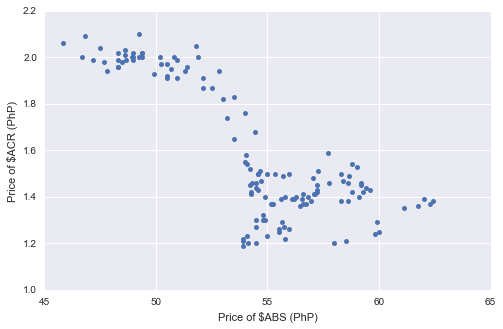In : ## FMETF and ALI plt.figure(figsize=(8,5)) plt.plot(final_df['FMETF'], final_df["ALI"], '.', markersize=10) plt.xlabel("Price of$FMETF (PhP)")
plt.ylabel("Price of $ALI (PhP)") _ = plt.show()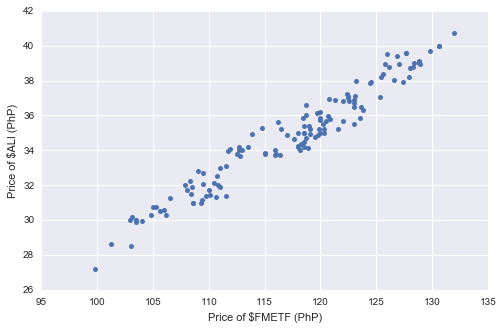In : ## BLOOM and BDO plt.figure(figsize=(8,5)) plt.plot(final_df['BLOOM'], final_df["BDO"], '.', markersize=10) plt.xlabel("Price of$BLOOM (PhP)")
plt.ylabel("Price of $BDO (PhP)") _ = plt.show()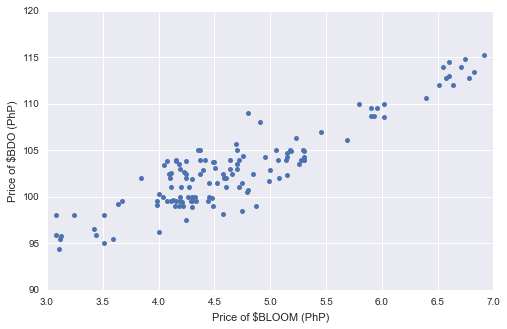Below, we draw the heatmap of the resulting correlation matrix. In : ## Source: https://stanford.edu/~mwaskom/software/seaborn/examples/many_pairwise_correlations.html ## Generate a mask for the upper triangle mask = np.zeros_like(price_corr, dtype=np.bool) mask[np.triu_indices_from(mask)] = True ## Set up the matplotlib figure f, ax = plt.subplots(figsize=(15, 15)) ## Generate a custom diverging colormap cmap = sns.diverging_palette(220, 10, as_cmap=True) ## Draw the heatmap with the mask and correct aspect ratio sns.heatmap(price_corr, mask=mask, cmap=cmap, vmax=.3, square=True, xticklabels=2, yticklabels=2, linewidths=.5, cbar_kws={"shrink": .5}, ax=ax) _ = plt.show()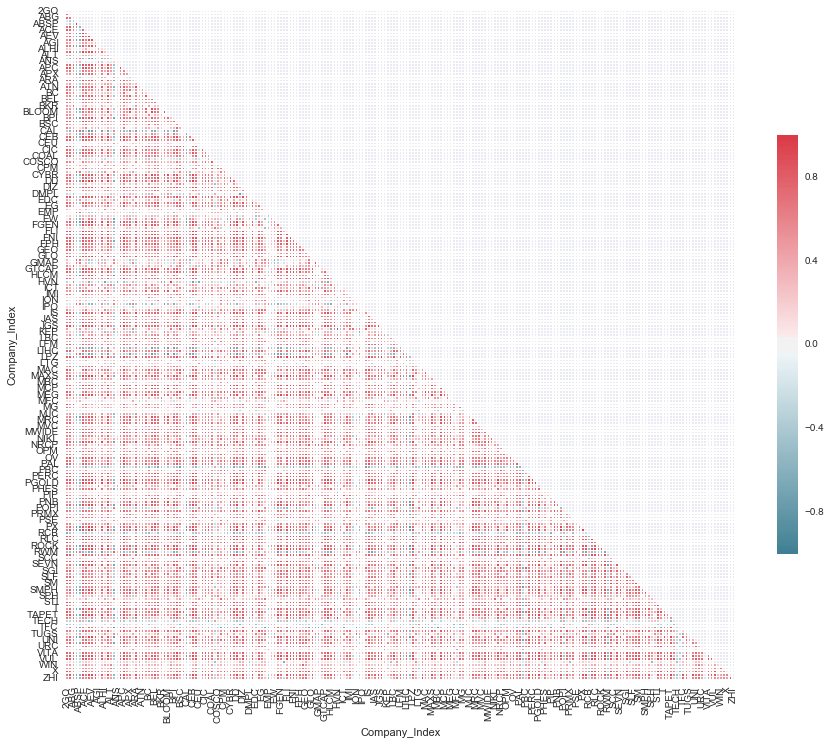### Step 2: Build Distance Matrix¶ As mentioned in the blog post, we will use two distance metrics in building the distance matrices. The first metric is from Bonanno et al. \begin{equation}d_{ij} = \sqrt{2 \times (1 - c_{ij})}\end{equation} where$c_{ij}$is the correlation cofficient of stocks$i$and$j$. In the equation, when$c_{ij}=1$,$d_{ij}=0$; and, when$c_{ij}=-1$,$d_{ij}=2$. That is, when there is a perfectly positive correlation (+1), the distance is 0; and, when the correlation is perfectly negative, the distance is the farthest at 2. The next distance measure is from SliceMatrix (mktstk) and it is given by \begin{equation}d_{ij} = 1 - |c_{ij}|.\end{equation} This equation does not distinguish between a positively or a negatively correlated pair of stocks; as long as two stocks are highly correlated, the distance is minimized. Here, we define the distance matrices as dist_bonanno and dist_mktstk. In : dist_bonanno = np.sqrt(2*(1-(price_corr))) dist_mktstk = 1-abs(price_corr) ## I am just defining the labels labs_bonanno = list(dist_bonanno.index) labs_mktstk = list(dist_mktstk.index)  ### Step 3: Build Minimum Spanning Tree (MST)¶ Now, we are ready to build the minimum spanning tree. The idea is to connect the ones that have the closest distance to each other, i.e. connect those that are highly correlated. Let's first build the "weighted" networks G_bonanno and G_mktstk from the distance matrices dist_bonanno and dist_mktstk, respectively. Using the Python package NetworkX, that's pretty straightforward to do. In : G_bonanno = nx.from_numpy_matrix(dist_bonanno.as_matrix()) G_mktstk = nx.from_numpy_matrix(dist_mktstk.as_matrix())  Once we have the distance networks, we can already build minimum spanning trees (MST). Here, we use Kruskal's algorithm. Below is the pseudo-code copied from the Wikipedia entry on the algorithm. KRUSKAL(G): 1 A = ∅ 2 foreach v ∈ G.V: 3 MAKE-SET(v) 4 foreach (u, v) in G.E ordered by weight(u, v), increasing: 5 if FIND-SET(u) ≠ FIND-SET(v): 6 A = A ∪ {(u, v)} 7 UNION(u, v) 8 return A  Again, we can use NetworkX to build the MST with the graphs as inputs. In : MST_b = nx.minimum_spanning_tree(G_bonanno) MST_m = nx.minimum_spanning_tree(G_mktstk)  Finally, let's add more attributes to the "nodes" or the stocks. The attributes that I want to include here are: • label (the stock symbol) • sector (which sector the stock belongs) • change (the$\%\$ change of the stock for the period under study)

This way, when we draw the MSTs, we can choose to color the nodes by either sector or change.

In :
change = (final_df.iloc[-1] - final_df.iloc) * 100 / final_df.iloc

In :
for node in MST_b.nodes():
sector = pse_companies[pse_companies["Stock Symbol"] == labs_bonanno[node]].Sector.iloc
MST_b.node[node]["sector"] = sector
MST_b.node[node]["label"] = labs_bonanno[node]
if math.isnan(change[labs_bonanno[node]]):
MST_b.node[node]["color"] = "black"
elif change[labs_bonanno[node]] < -10:
MST_b.node[node]["color"] = "red"
elif change[labs_bonanno[node]] > 10:
MST_b.node[node]["color"] = "green"
else:
MST_b.node[node]["color"] = "blue"

In :
for node in MST_m.nodes():
sector = pse_companies[pse_companies["Stock Symbol"] == labs_mktstk[node]].Sector.iloc
MST_m.node[node]["sector"] = sector
MST_m.node[node]["label"] = labs_mktstk[node]
if math.isnan(change[labs_mktstk[node]]):
#print change[labs_mktstk[node]], labs_mktstk[node]
#Gm.node[node]["change"] = 101
MST_m.node[node]["color"] = "black"
elif change[labs_mktstk[node]] < -10:
MST_m.node[node]["color"] = "red"
elif change[labs_mktstk[node]] > 10:
MST_m.node[node]["color"] = "green"
else:
MST_m.node[node]["color"] = "blue"


### Drawing the MSTs¶

In :
plt.figure(figsize=(10,10))
nx.draw_networkx(MST_b)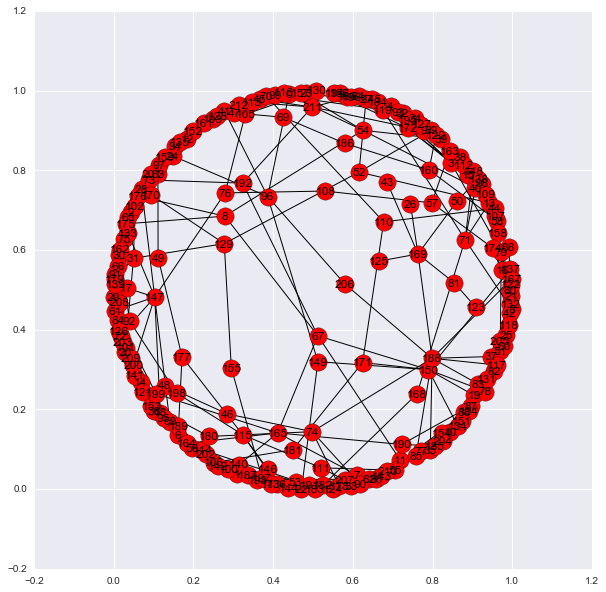In :
plt.figure(figsize=(10,10))
nx.draw_networkx(MST_m)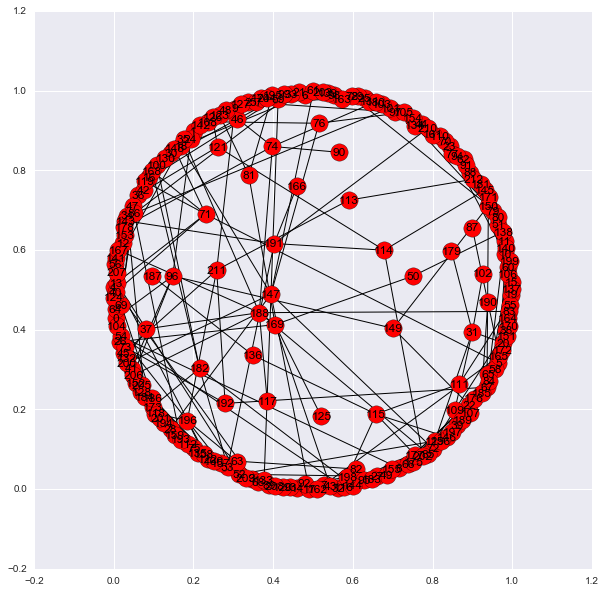### Write out MSTs¶

Below, we write the MSTs as gexf files so we can use them in Gephi (open-source and free) to generate much prettier networks/trees.

Gephi is the leading visualization and exploration software for all kinds of graphs and networks. Gephi is open-source and free.
In :
nx.write_gexf(MST_b, "corrmat_bonanno.gexf")
nx.write_gexf(MST_m, "corrmat_mktstk.gexf")


Below is the resulting network (MST_b) drawn using Gephi.In [ ]: